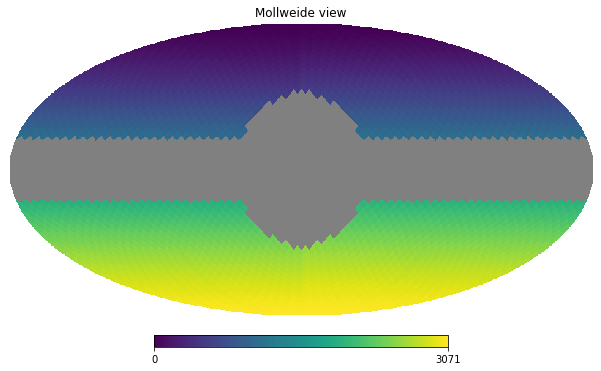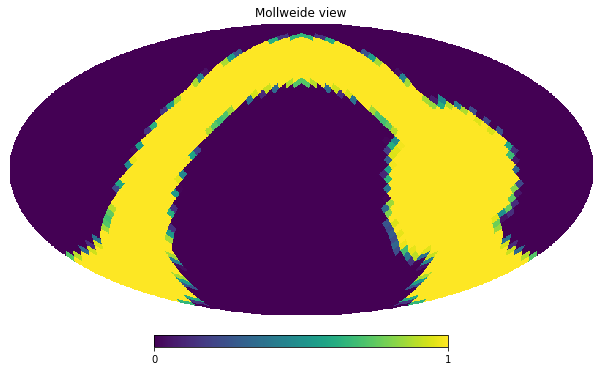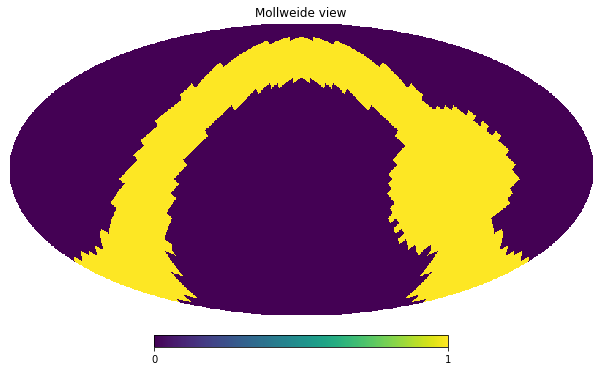import healpy as hp
import numpy as np
%matplotlib inline

hp.disable_warnings()

nside = 16
npix = hp.nside2npix(nside)

m = hp.ma(np.arange(npix, dtype=np.float32))

mask = np.zeros(npix, dtype=np.bool)

m.mask = mask

hp.mollview(m);gal2eq = hp.Rotator(coord=["G","E"])

m_rotated = gal2eq.rotate_map_pixel(m)

mask_rotated = gal2eq.rotate_map_pixel(m.mask)

hp.mollview(mask_rotated)hp.mollview(m_rotated.mask)Now in the first case healpy fills the map with UNSEEN and then interpolation is handled by HEALPix C++. I don't know how internally HEALPix handles that. In the second case we pass a map of 0 and 1 and HEALPix does the interpolation, but we don't trigger any special case of handling UNSEEN values.

np.testing.assert_array_equal(m_rotated.mask, mask_rotated == 1)

---------------------------------------------------------------------------
AssertionError                            Traceback (most recent call last)
<ipython-input-13-aa7e79abeb94> in <module>
1 # consider all values less than 1 masked

[... skipping hidden 2 frame]

AssertionError:
Arrays are not equal

Mismatched elements: 105 / 3072 (3.42%)
x: array([False, False, False, ..., False, False, False])
y: array([False, False, False, ..., False, False, False])
np.testing.assert_array_equal(m_rotated.mask, mask_rotated > 0)

---------------------------------------------------------------------------
AssertionError                            Traceback (most recent call last)
<ipython-input-14-47376e435ed3> in <module>
1 # consider only values of 1 masked

[... skipping hidden 2 frame]

AssertionError:
Arrays are not equal

Mismatched elements: 169 / 3072 (5.5%)
x: array([False, False, False, ..., False, False, False])
y: array([False, False, False, ..., False, False, False])
np.testing.assert_array_equal(m_rotated.mask, mask_rotated > .9)

---------------------------------------------------------------------------
AssertionError                            Traceback (most recent call last)
<ipython-input-15-acaca61476b4> in <module>
1 # try a value close to 1

[... skipping hidden 2 frame]

AssertionError:
Arrays are not equal

Mismatched elements: 26 / 3072 (0.846%)
x: array([False, False, False, ..., False, False, False])
y: array([False, False, False, ..., False, False, False])
np.testing.assert_array_equal(m_rotated.mask, mask_rotated > .999)

---------------------------------------------------------------------------
AssertionError                            Traceback (most recent call last)
<ipython-input-16-7f299a009890> in <module>
1 # try a value close to 1
y: array([False, False, False, ..., False, False, False])
np.testing.assert_array_equal(m_rotated.mask, mask_rotated > .9999)